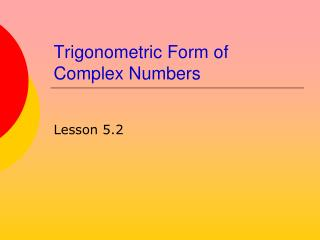DownloadDownload PresentationTrigonometric Form of Complex Numbers

# Trigonometric Form of Complex Numbers

Download Presentation## Trigonometric Form of Complex Numbers

- - - - - - - - - - - - - - - - - - - - - - - - - - - E N D - - - - - - - - - - - - - - - - - - - - - - - - - - -
##### Presentation Transcript

1. Trigonometric Form of Complex Numbers Lesson 5.2

2. Graphical Representation of a Complex Number • Graph in coordinate plane • Called the complex plane • Horizontal axisis the real axis • Vertical axis is the imaginaryaxis 3 + 4i• -2 + 3i• • -5i

3. Absolute Value of a Complex Number • Defined as the length of the line segment • From the origin • To the point • Calculated byusing PythagoreanTheorem 3 + 4i•

4. Find That Value, Absolutely • Try these • Graph the complex number • Find the absolute value

5. Trig Form of Complex Number • Consider the graphical representation • We note that a righttriangle is formed a + bi• r b θ a How do we determine θ?

6. Trig Form of Complex Number • Now we use and substitute into z = a + bi • Result is • Abbreviation is often

7. Try It Out • Given the complex number -5 + 6i • Write in trigonometric form • r = ? • θ = ? • Given z = 3 cis 315° • Write in standanrd form • r = ? • a = ? • b = ?

8. Product of Complex Numbers in Trig Form • Given • It can be shown that the product is • Multiply the absolute values • Add the θ's

9. Quotient of Complex Numbers in Trig Form • Given • It can be shown that the quotient is

10. Try It Out • Try the following operations using trig form • Convert answers to standard form

11. Assignment • Lesson 5.2 • Page 349 • Exercises 1 – 61 EOO